# Fighting with high-dimensional not-so-small data

Vadim Markovtsev - Codemotion Madrid, 2017

Vadim Markovtsev

Fits 1 node
≥ 2 nodes
Casual
Bleeding edge
easy, fast
easy, slow
easy, slow
hard, slow

## How to determine what's your size?

• Is your data sparse or can be made sparse?
• Does the processing fit into 256/512GB?
• Does it take less than 1 hour/day/week?
• Do you expect that it will not in the future?

## How to determine what's your complexity?

Source
System complexity
← Modeling difficulty
Easy to model
Simple domain
Difficult to model
Simple domain
Easy to model
Complex domain
Difficult to model
Complex domain

Vertical
Horizontal
Casual
Bleeding edge
useless
required
easy
hard

## The case of Netflix• Sparse-aware
• IO agnostic
• Single-machine

## Approaches

• PCA
• DSSM
• CUR
• t-SNE
• Topic Modeling
• LargeVis, UMAP
• *2vec

## PCA

• The lowered number of dimensions depends on the explained variance ratio (EVR).
• EVR =
Σλ2trace(XTX)
Source

## PCA

• Centers the columns.
• Does not work with sparse matrices.
• Output is never sparse.

## PCA

• Unstable to outliers.
• Possible solutions: filtering; log or any other damping function.

## Truncated SVD

• The efficient method to calculate PCA.
• PCA(X, N) = TSVD(centered(X), N).
• Supports sparse input.
• Output is never sparse.

## Proven implementations

• fbpca - approximate, large scale.
• sklearn - exact and approximate, small and medium scale.

## CUR

• Randomized, approximate TSVD.
• Supports sparse input.
• Output is sparse.
• Vulnerable to outliers.
• Implementation: PyMF.

## Topic Modeling

• Family of unsupervised models to mine sets of probabilistically common subsets from a series of sets.
• Example: topics in text documents.
• Those sets must be labeled by humans.
• Implementations: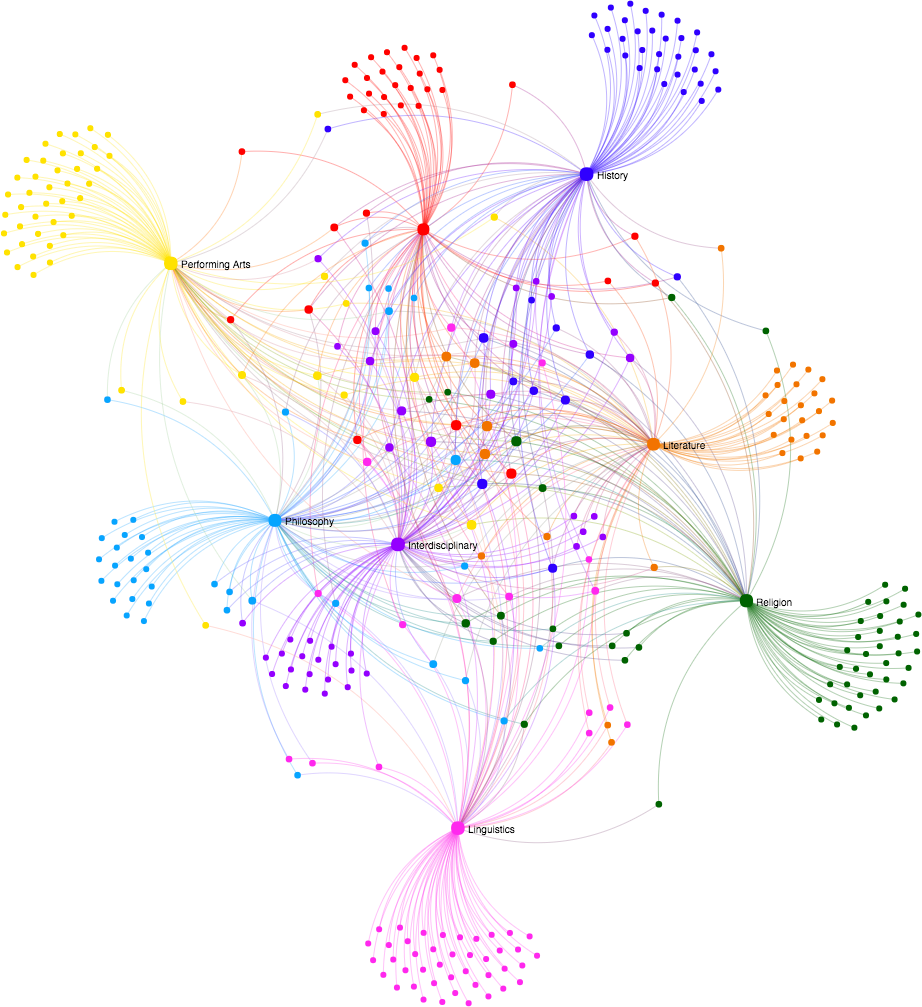Source

## Topic Modeling

• Topics are not clusters!
• Good for explaining, bad for decisions.## Sequential data

• We are surrounded by sequential data:
• Texts, system logs, etc.
• Usenet dataset example:
• Moderate size: ~7B words ~37GB.
• A lot of unique entities.

## Embeddings

### Properties:

• Low-dimensional representation.
• Similar contexts -> similar embeddings.
• Analogies search.
• Reasoning: W("woman") − W("man") ≃ W("queen") − W("king").

## word2vec - skip-gram

• Predicting the context given the word:
• Input: "mcube".
• Output: "is", "a", "great", "conference".
• Works well even with small data.
• Represents well rare words.

## word2vec - CBOW

• Predicting the word given its context.
• Input: "is", "a", "great", "conference".
• Output: "mcube".
• Faster training than the skip-gram.
• Better accuracy for the frequent words.

## word2vec - fastText

• Embeddings for char n-grams.
• Emb(word)= Σ Emb(char n-gram)
-> embeddings for unseen words.
• Classification of texts.
• Compression of models.

## Embeddings - global vectors

Approach combines the advantages of the two major model families:

• Global matrix factorization.
• Local context window.

## Embeddings - global vectors

Example:

• "Text with 5 unique words".
• Window size: 2.

## Swivel

Embedding engine which works on co-occurrence matrices.

Like GloVe, but better - Paper on ArXiV.

We forked the Tensorflow implementation.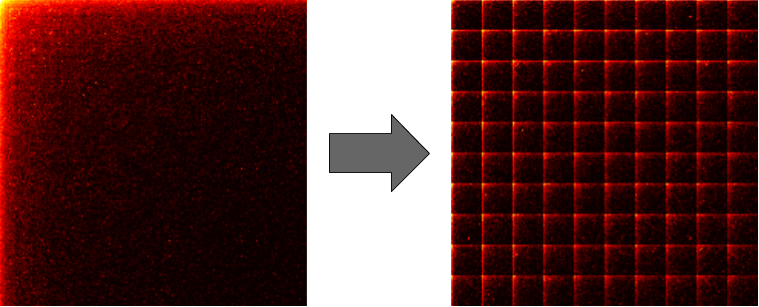## Swivel

• Features:
• Multi-GPU support.
• Scales with vocabulary size.
• Efficient sharding algorithm to distribute workload.

10s~100s GBs
100s~ GBs
~1M uniq
many M uniq
CBOW
skip-gram
Swivel
bad luck

## Graph embeddings

Problems

• Social graphs, dependency graphs, etc.

Properties:

• Continuous feature representations for nodes in networks.
• Maximizes the likelihood of of preserving network neighborhoods of nodes.

## node2vec

Approach:

• Random walks over a graph - gives sequences of nodes.
• Sequences of nodes - stochastic approximation of graph.
• Use these sequences as input to word2vec / Swivel / etc. -> embeddings.
• Result: embeddings for nodes.

## node2vec

• n1 -> n1025 -> n37 ...
• n2 -> n53 -> n209 ...
• n3 -> n129 -> n548 ...
• n4 -> n78 -> n789 ...
• ...

## The case of Facebook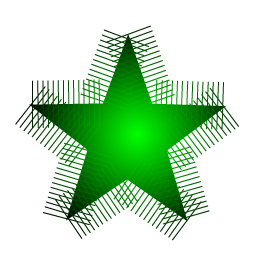• Starspace
• Word, sentence, document, graph embeddings.
• Text classification, ranking.
• CF and Content recommendation.
• Faiss
• Dense k-NN.
• Devilish GPU implementation.

## Deep semantic similarity model

• Article.
• Term vector - 500k unique words.
• 3-grams of characters (30k):
• #bad# -> #ba, bad, ad#
• Deep representation.
• Cosine similarity.

## Data visualization

Task definition:

• We are given a collection of high-dim data.
• How can we get a feel for how these objects are arranged in space?

## t-SNE

Why was it introduced?

• Distance preservation is not enough.
• We often care about the distance
relations, not exact values.

## t-SNE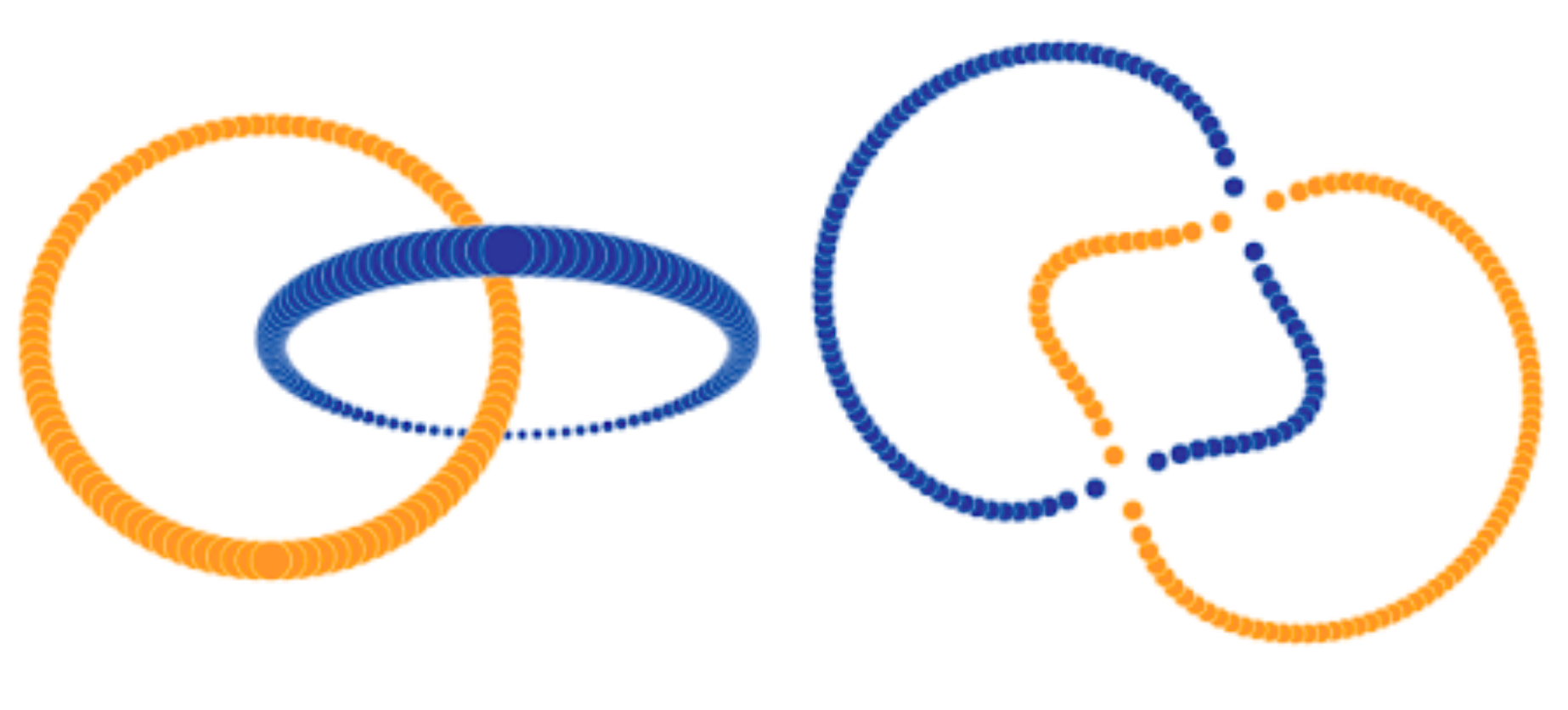• Preserves the neighborhood.
• Respects the relation between distances and not the exact values.
• Works with N dimensions ~102, requires reduction if more.
• Complexity: exact O(d*N2) & Barnes-Hut O(d*N*log(N)).
• Excellent blog post.

## LargeVis

• Large scale t-SNE replacement. GitHub
• Complexity: O(s*D*N).

## Uniform Manifold Approximation and Projection

• Large scale t-SNE replacement (2). GitHub
• Complexity: O(D*N1.14).
• Can also be applied as
a non-linear dimension reduction.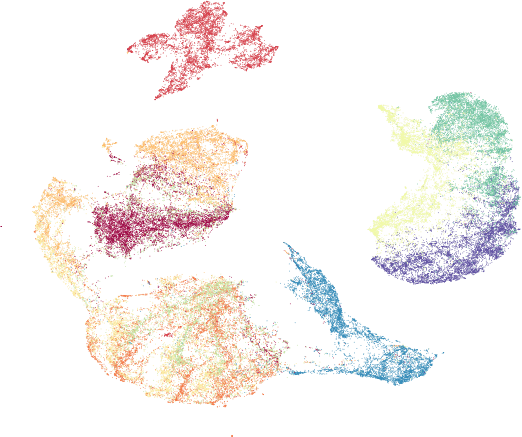## Conclusion

• Fit data into single node.
• Reduce the amount of data.
• Try a sparse-friendly model.
• Eliminate outliers.
• PCA can be good for pre-processing.
• Embed and conquer.
• Visualize early.